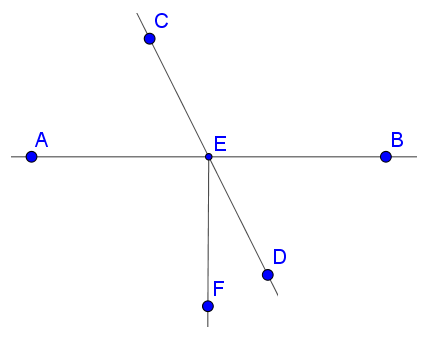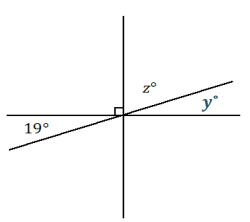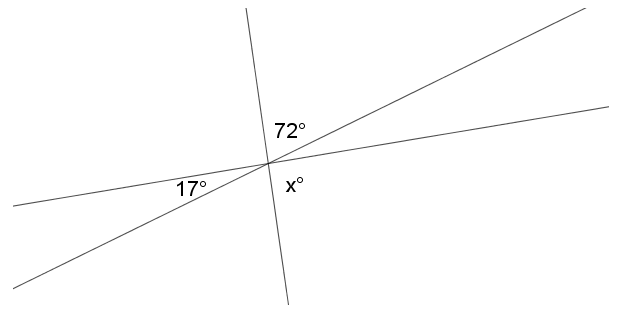Match Fishtank is now Fishtank Learning!

# Geometry

## Objective

Use vertical, complementary, and supplementary angle relationships to find missing angles.

## Common Core Standards

### Core Standards

?

• 7.G.B.5 — Use facts about supplementary, complementary, vertical, and adjacent angles in a multi-step problem to write and solve simple equations for an unknown angle in a figure.

?

• 4.MD.C.5

• 4.MD.C.6

• 4.MD.C.7

## Criteria for Success

?

1. Understand that vertical angles are the pair of angles formed across from one another when two lines intersect, and that the measurements of vertical angles are congruent.
2. Identify pairs of vertical angles in angle diagrams.
3. Find the values of angles using vertical, complementary, and supplementary angle relationships and equations.

## Tips for Teachers

?

The following materials are needed for this lesson: protractors and rulers.

#### Remote Learning Guidance

If you need to adapt or shorten this lesson for remote learning, we suggest prioritizing Anchor Problem 1 (benefits from worked example). As a discovery problem, if live discussion or reflection of the problem were possible, it would allow for students to arrive at the conclusion on their own. Find more guidance on adapting our math curriculum for remote learning here.

#### Fishtank Plus

• Problem Set
• Student Handout Editor
• Vocabulary Package

## Anchor Problems

?

### Problem 1

In small groups, draw two intersecting lines on a piece of paper.

• Label the angles formed by the two lines as 1–4, in a clockwise direction.
• Measure each angle with a protractor and record the information.
• Compile your results as a class.

Vertical angles are a pair of angles formed across from one another when two lines intersect. Based on the data from your class, what can you conclude about the measurements of vertical angles?

### Problem 2

In the diagram below, line ${CD}$  intersects line ${AB}$  through point $E$. Ray $EF$  extends from point $E$.Callie says that $\angle CEB$ is vertical to $\angle AEF$. Explain why her reasoning is incorrect and name the angle that is vertical to $\angle CEB$.

### Problem 3

Three lines meet at a point, as shown below.1. Describe the angle relationships you see in the diagram.
2. Set up and solve an equation to find the value of $z$.

#### References

EngageNY Mathematics Grade 7 Mathematics > Module 6 > Topic A > Lesson 2Example 2

Grade 7 Mathematics > Module 6 > Topic A > Lesson 2 of the New York State Common Core Mathematics Curriculum from EngageNY and Great Minds. © 2015 Great Minds. Licensed by EngageNY of the New York State Education Department under the CC BY-NC-SA 3.0 US license. Accessed Dec. 2, 2016, 5:15 p.m..

Modified by Fishtank Learning, Inc.

## Problem Set

?The following resources include problems and activities aligned to the objective of the lesson that can be used to create your own problem set.

?

Three lines intersect at a common point, as shown in the diagram below.Write and solve an equation to determine the value of $x$.

?